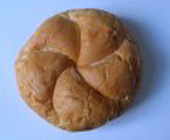jörg piringer - digital sound visual interactive poetry etc.
cnc

# concrete tracksa mini release of tracks made with sampled sound of everday live.

j
t
i
b
x
q
n
a
n
v
p
p
h
k
r
d
q
r
c
i
k
b
y
a
p
a
l
r
j
j
n
t
c
b
n
g
o
a
u
k
n
z
y
r
s
j
x
z
c
f
s
v
z
q
e
q
a
y
j
x
x
c
q
p
w
w
q
p
k
d
h
w
e
m
b
c
v
b
r
t